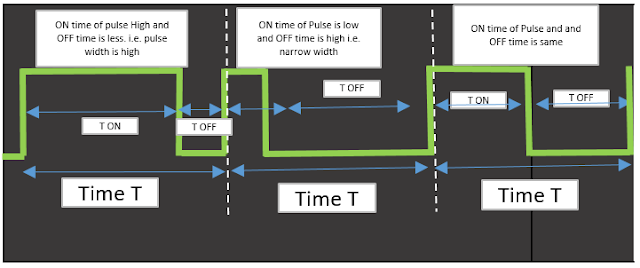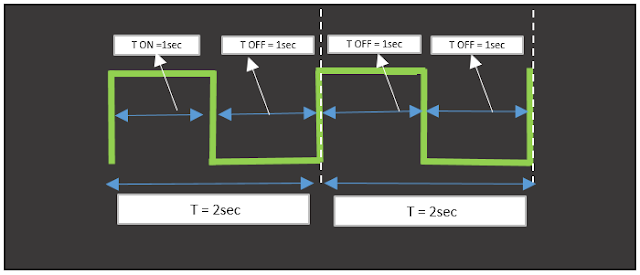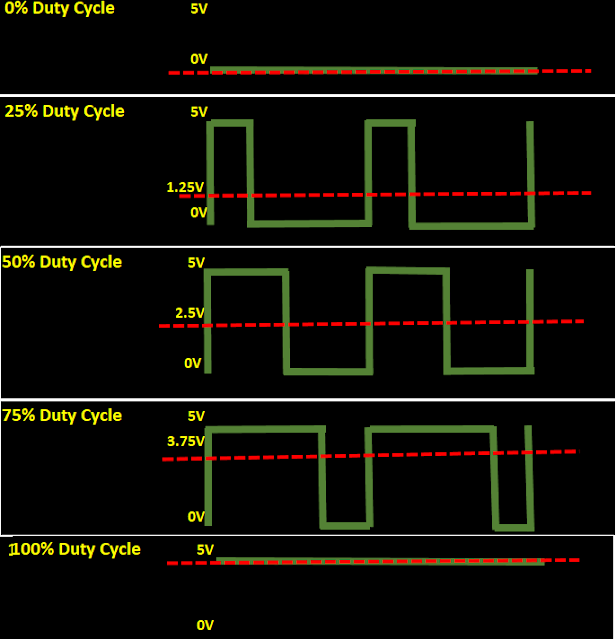# | PWM (Pulse Width Modulation) in Embedded System

0

1. What is PWM
2. What is duty cycle , TON and TOFF
3. How to calculate TON and TOFF based on duty cycle
4. How to generate PWM using timers
5. Applications and need of PWM
6. Average voltage of PWM wave calculation formula.

## 1. Introduction to PWM :

Full form of PWM is pulse width modulation, i.e. in PWM width of the pulse is varied by keeping period of pulse constant.

As shown in below image width of pulse is varied but time T is constant.Fig. 1  PWM Concept

Now lets go into more details. PWM pulse has ON time i.e. TON and OFF time i.e. TOFF. ON time is time for which PWM pulse is high and OFF Time is time for which PWM pulse is low. In PWM we can change time duration of TON or TOFF and hence width of the pulse is varied but period of pulse is constant i.e. T(Period of pulse)= TON+TOFF

Consider PWM pulse with  TON=1 sec and TOFF =1sec. Period of Pulse is TON+TOFF = 1sec +1sec = 2sec. i.e. frequency of PWM pulse is 0.5 Hz

Now you can change TON and TOFF like :
TON  = 1.5 sec and TOFF=0.5 sec  by keeping period of pulse unchanged i.e. T=2sec
TON  = 0.5 sec and TOFF=1   sec.  by keeping period of pulse unchanged i.e. . T=2sec

###Fig.2 PWM with TON 1sec and TOFF 1sec1.1 What is Duty Cycle :

Now variation of TON and TOFF can be measured by duty cycle :
Duty cycle(%)  = (TON/(TON+TOFF))*100
i.e. duty cycle represents, for how much time pulse is high, out of total pulse period.

So ,
50% duty cycle means TON= 1sec    and TOFF = 1sec
75% duty cycle means TON= 1.5sec  and TOFF = 0.5 sec
25% duty cycle means TON= 0.5 sec and TOFF = 1.5sec

### 1.2 Calculation of TON and TOFF based on Duty Cycle :

Consider above example, PWM with frequency of 0.5Hz and duty cycle is 10% then
10% duty cycle means TON is for 10% period and TOFF is for 90% period

i.e.  10%2 sec = 10/100*2 sec = 0.2sec TON
90%2 sec = 90/100*2 sec = 0.8sec TOFF

TON = 0.2sec and TOFF= 0.8sec

### 1.3 What is frequency of PWM :

Frequency of PWM is calculated as Fpwm = 1/(TON+TOFF).

# 2. What is use of PWM :

In previous section you have learnt what  is PWM, duty cycle and frequency of PWM.  In this section we will learn what is use of PWM and what is importance of duty cycle and importance of PWM frequency .

PWM is used to control analog circuit digitally (as PWM is digital)  Now in real life you can control  LED brightness using PWM. But how ?

Consider Fig.3 which shows a micro-controller connected to LED. PIN to which LED connected is having PWM functionality i.e. output of PIN is PWM. Now we will see how brightness of LED is controlled by PWM.

PWM is digital pulse and lets consider  voltage of PWM is 5V or 0V i.e. digital 1 or digital 0 (Vout of PIN is 5V or 0V). Consider 0% duty cycle, 25% duty cycle,50% duty cycle and 100% duty cycle, as shown in figure 3.Fig.3  PWM Duty Cycle and Average Output voltage

By applying PWM pulse to LED, an Average Voltage is applied to LED.  Average voltage of PWM wave is shown by dotted line.  Average Voltage :

Vout(Average Voltage) = Vmax*duty cycle.
Vmax is 5V (maximum output voltage of MCU Pin)

PWM duty cycle kept as    0%  then Vout (Average Voltage) = 5*0/100      = 0V
PWM duty cycle kept as  25%  then Vout (Average Voltage) = 5*25/100    = 1.25V
PWM duty cycle kept as  50%  then Vout (Average Voltage) = 5*50/100    = 2.5V
PWM duty cycle kept as  75%  then Vout (Average Voltage) = 5*75/100    = 3.7.5V
PWM duty cycle kept as 100% then Vout (Average Voltage) = 5*100/100  = 5V

LED brightness can be controlled using PWM by varying duty cycle i.e. by varying average out voltage.

Consider PWM as switch, for TON , LED will be turn on (as LED will receive 5V) and For TOFF(as LED will receive 0V) LED will be turn off. If you keep TON as 1 sec and TOFF as 1 sec then you will observe ON and OFF of LED. You will never observe LED dimming effect i.e. changing brightness LOW to High, this is due to PWM frequency is low.

To do so, we need to apply PWM pulse to LED with high PWM frequency, so that ON and OFF state of LED will change so fast. So if you change frequency of output pulse and keep it as 1Khz i.e. Period of Pulse is 1ms and duty cycle of PWM is 50%  then for 0.5ms LED will off and 0.5ms LED will be on and this would happen so fast that dimming effect will occur as LED receives only 2.5V

if you change the duty cycle to 10% then LED will have low brightness , as it will be OFF for 90% of time and ON for 10% time.

if you change the duty cycle to 90% then LED will be more brighter, as it will be ON for 90% time and OFF for 10% time.

Similar way you can control motor speed using PWM.

#### 2.1. Why PWM :

By Controlling analog circuit digitally system cost and power goes down.

# 3. Ways to generate PWM :

1st way : Most of micro-controller manufactures have started providing PWM as peripheral so that it would be easy to generate it. Using PWM peripheral i.e. on pin of micro-controller is set to PWM output, by setting duty cycle and frequency of PWM, MCU will calculate TON and TOFF and generates required PWM pulse on that pin.

2nd way :Also if MCU does not have PWM as peripheral there is a way to generate PWM pulse using timer. If you are not aware about timers the please read timer in embedded system :

https://www.embeddedtutor.com/2019/02/timercounter-in-embedded-system.html

how to generate PWM using timer?

1. Configure 2 timers. 1st timer is for TON and 2nd timer is for TOFF. (Values of TON and TOFF are depends on value of duty cycle and frequency of PWM , as described in above sections)
2. Start TON timer (with TON time of pulse) once timer reaches to certain time value, stop 1st timer and start 2nd timer (with TOFF time of pulse). This can be done by using ISR of timers. Once timer 1 ISR executed, start Timer 2 and in timer 2 ISR start timer 1
3. When 1st timer is started. set digital out pin to High (logic 1) and when 2nd timer started set same digital out pin to Low (logic 0).

Tags